【Go语言绘图】图片添加文字（一）

绘制纯色背景

package main

import "github.com/fogleman/gg"

func main() {
const S = 1024
dc := gg.NewContext(S, S)
dc.SetRGB(0, 1, 1)
dc.Clear()
dc.SavePNG("out.png")
}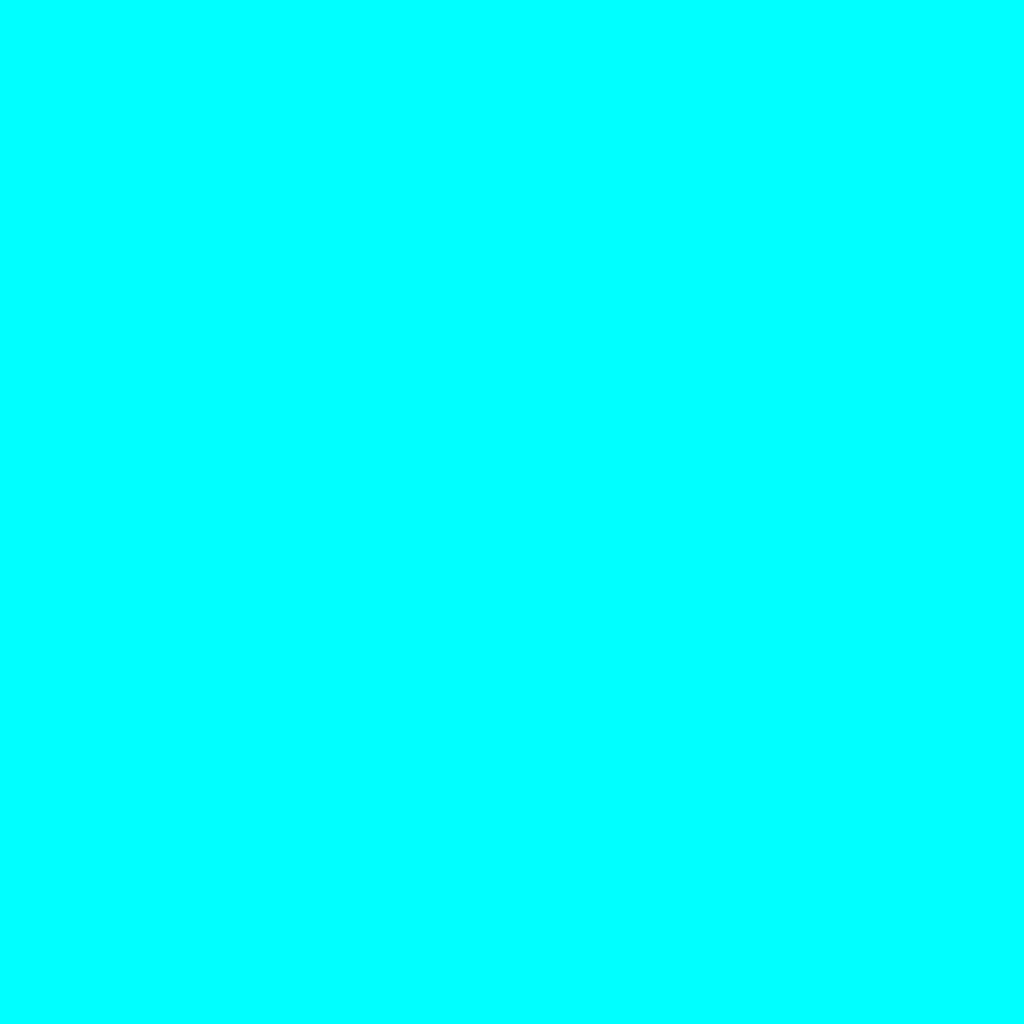func TestRotateImage(t *testing.T) {
width := 1000
height := 1000

dc := gg.NewContext(width, height)
dc.DrawRectangle(0, 0, float64(width), float64(width))
dc.SetRGB255(255, 255, 0)
dc.Fill()
dc.SavePNG("test.png")
}

// Clear fills the entire image with the current color.
func (dc *Context) Clear() {
src := image.NewUniform(dc.color)
draw.Draw(dc.im, dc.im.Bounds(), src, image.ZP, draw.Src)
}

添加文字

package main

import "github.com/fogleman/gg"

func main() {
const S = 1024
dc := gg.NewContext(S, S)
dc.SetRGB(0, 1, 1)
dc.Clear()
dc.SetRGB(0, 0, 0)
if err := dc.LoadFontFace("gilmer-heavy.ttf", 120); err != nil {
panic(err)
}
dc.DrawString("Hello, world!", 0, S/2)
dc.SavePNG("out.png")
}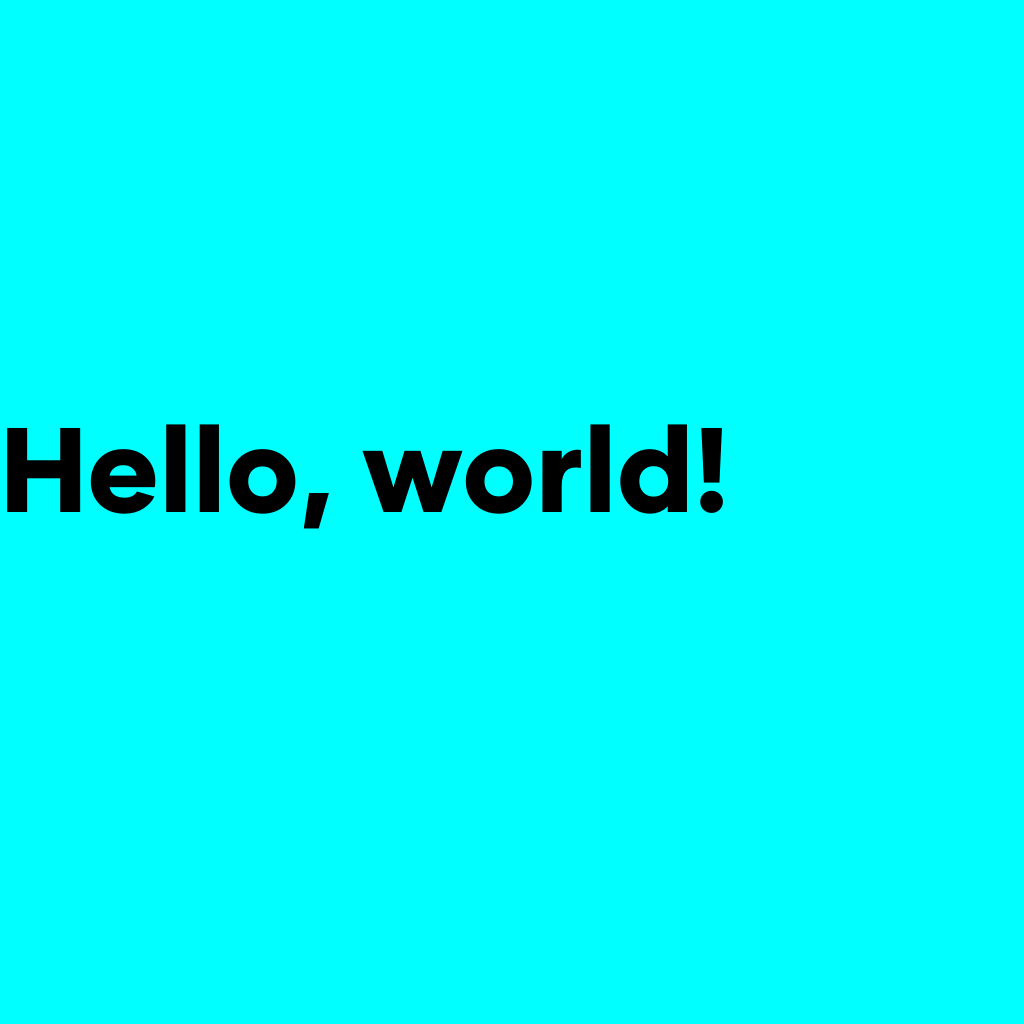dc.SetRGB(0, 0, 0)

if err := dc.LoadFontFace("gilmer-heavy.ttf", 120); err != nil {
panic(err)
}

func (dc *Context) LoadFontFace(path string, points float64) error {
if err == nil {
dc.fontFace = face
dc.fontHeight = points * 72 / 96
}
return err
}

func LoadFontFace(path string, points float64) (font.Face, error) {
if err != nil {
return nil, err
}
f, err := truetype.Parse(fontBytes)
if err != nil {
return nil, err
}
face := truetype.NewFace(f, &truetype.Options{
Size: points,
// Hinting: font.HintingFull,
})
return face, nil
}

调整字体大小

if err := dc.LoadFontFace("gilmer-heavy.ttf", 240); err != nil {
panic(err)
}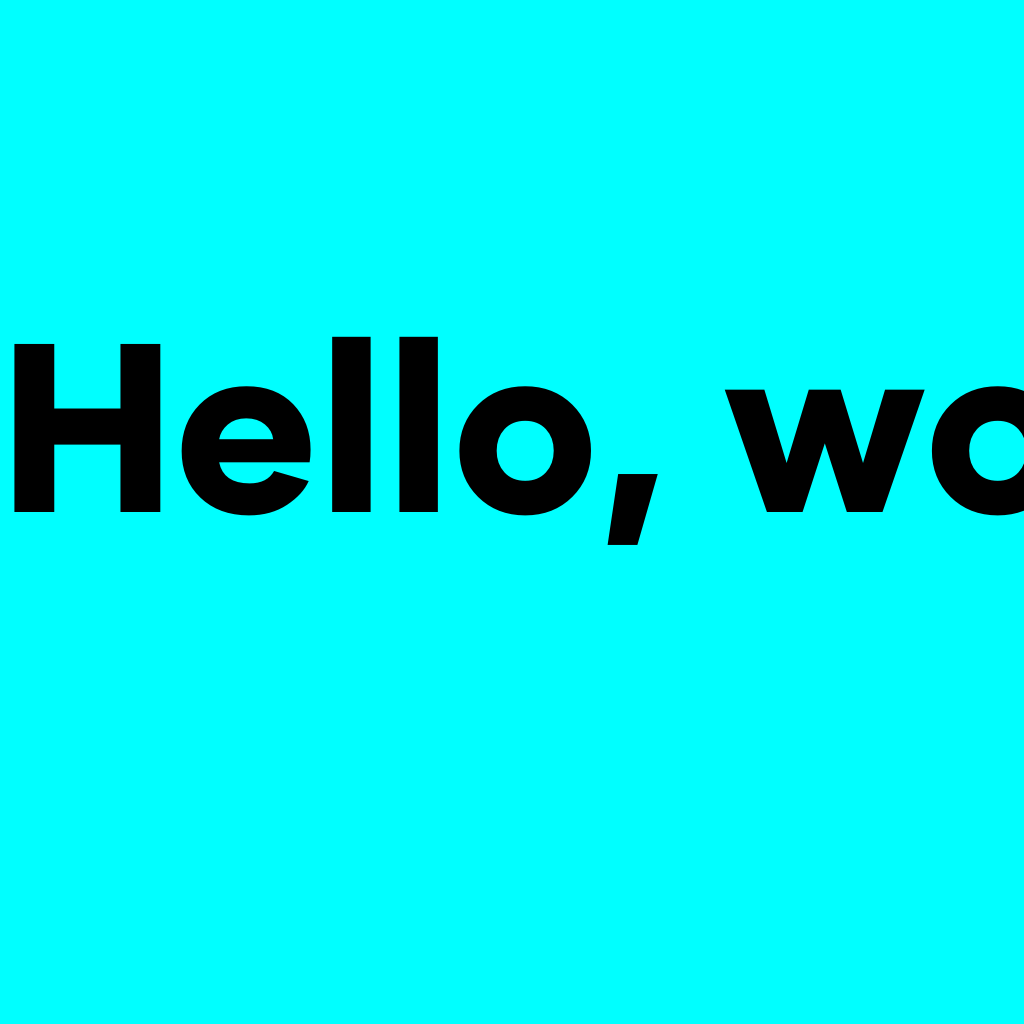居中显示

if err := dc.LoadFontFace("gilmer-heavy.ttf", 120); err != nil {
panic(err)
}
dc.DrawString("Hello, world!", 130, S/2)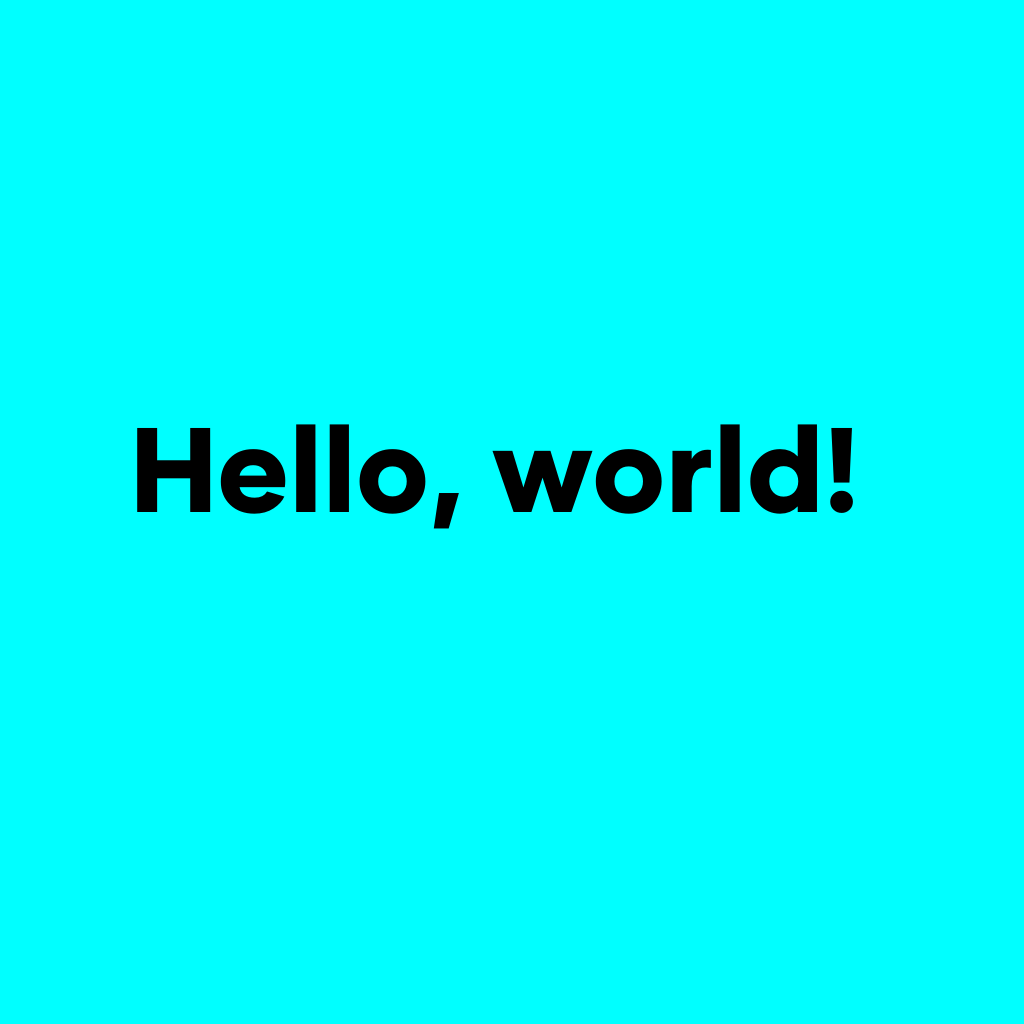s := "Hello, world!"
sWidth, sHeight := dc.MeasureString(s)
dc.DrawString(s, (S-sWidth)/2, (S+sHeight)/2)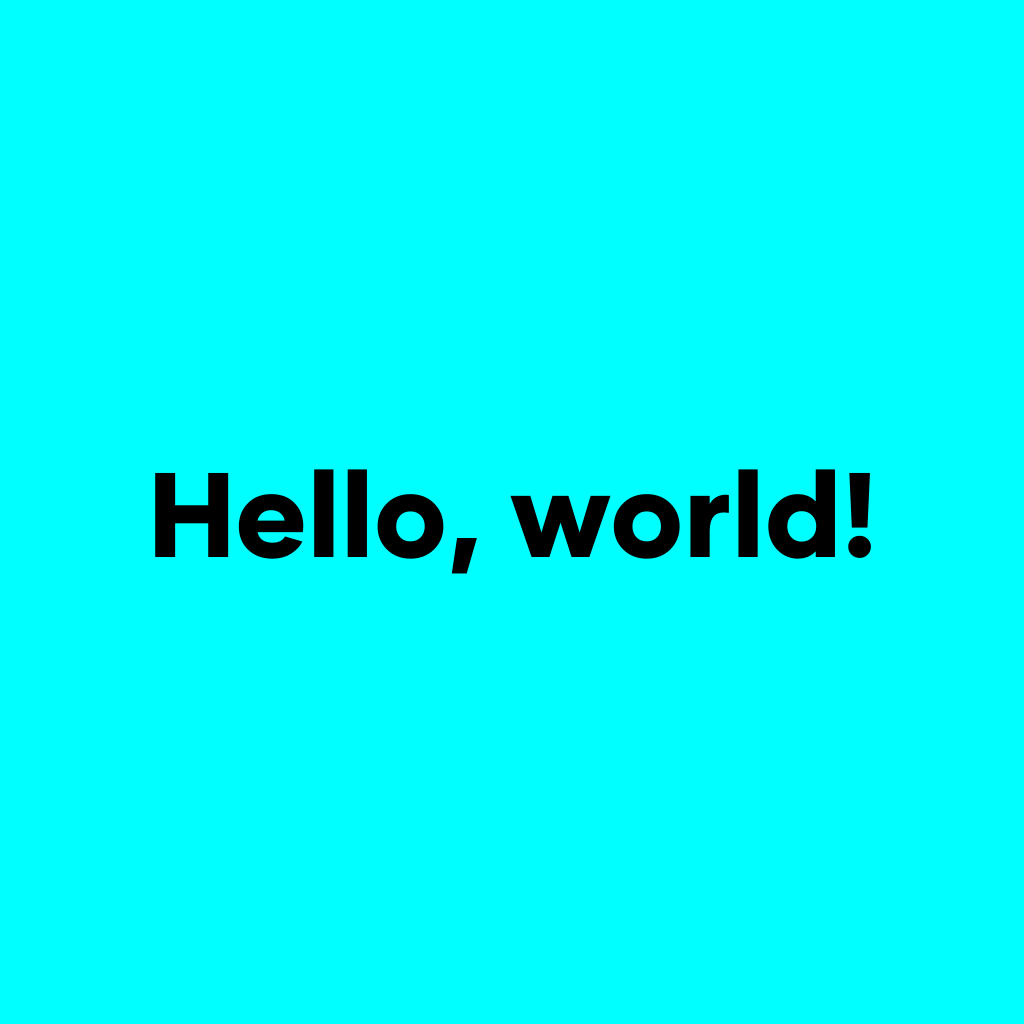s := "Hello,world! Hello,ByteDancer!"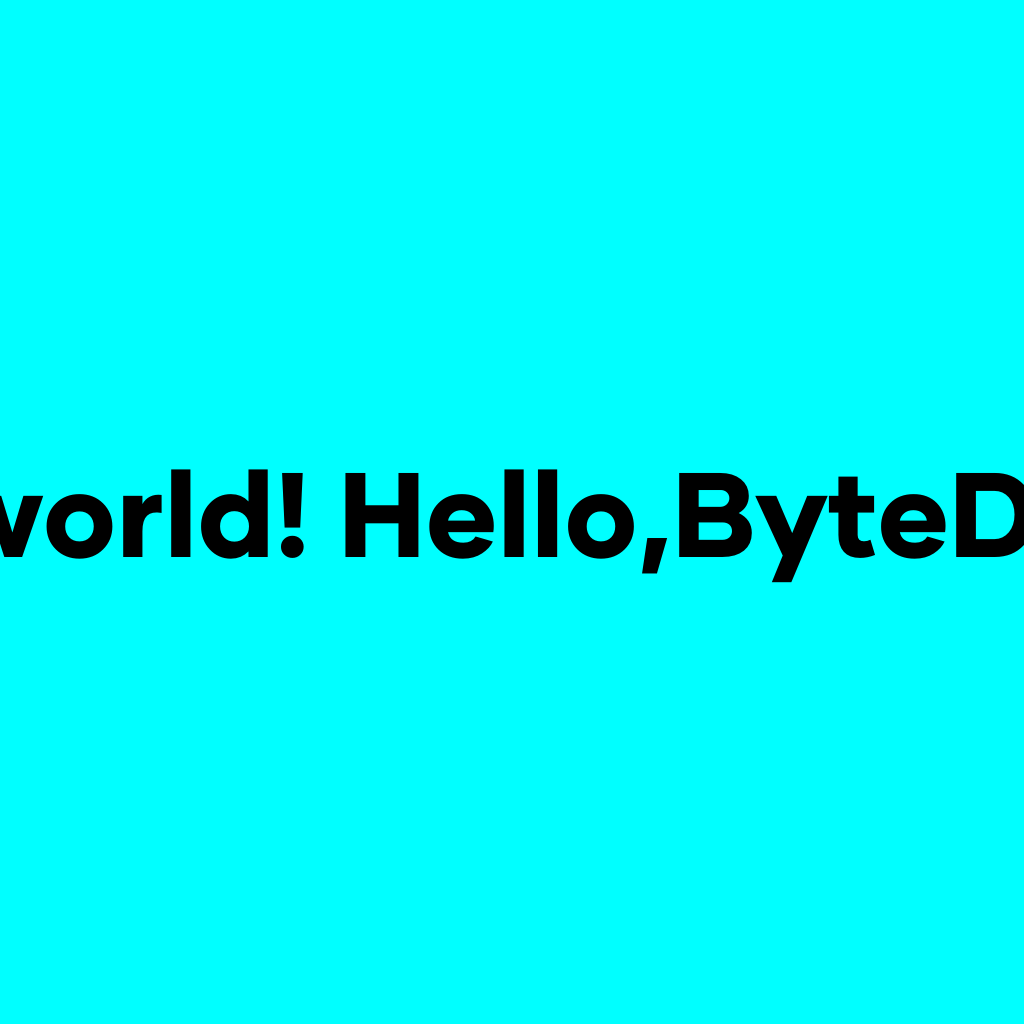单行长文本处理

maxTextWidth := S * 0.75

func TruncateText(dc *gg.Context, originalText string, maxTextWidth float64) string {
tmpStr := ""
for i := 0; i < len(originalText); i++ {
tmpStr = tmpStr + string(originalText[i])
w, _ := dc.MeasureString(tmpStr)
if w > maxTextWidth {
return tmpStr[0 : i-1]
}
}
return tmpStr
}

func main() {
const S = 1024
dc := gg.NewContext(S, S)
dc.SetRGB(0, 1, 1)
dc.Clear()
dc.SetRGB(0, 0, 0)
if err := dc.LoadFontFace("gilmer-heavy.ttf", 120); err != nil {
panic(err)
}
s := "Hello,world! Hello,ByteDancer!"
ellipsisWidth, _ := dc.MeasureString("...")
maxTextWidth := S * 0.75
s = TruncateText(dc, s, maxTextWidth - ellipsisWidth) + "..."
fmt.Println(s)
sWidth, sHeight := dc.MeasureString(s)

dc.DrawString(s, (S-sWidth)/2, (S+sHeight)/2)
dc.SavePNG("out.png")
}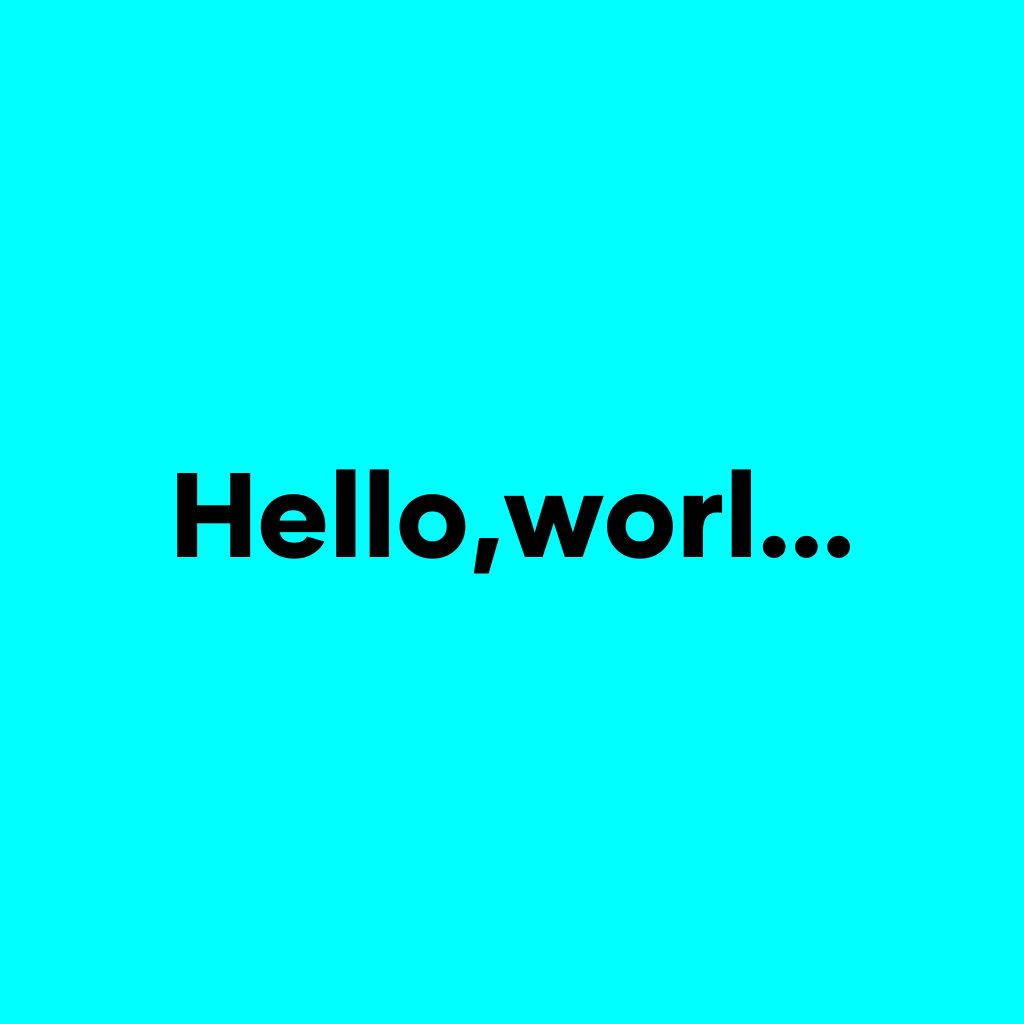if err := dc.LoadFontFace("方正楷体简体.ttf", 120); err != nil {
panic(err)
}
s := "如果我们把文字换成中文"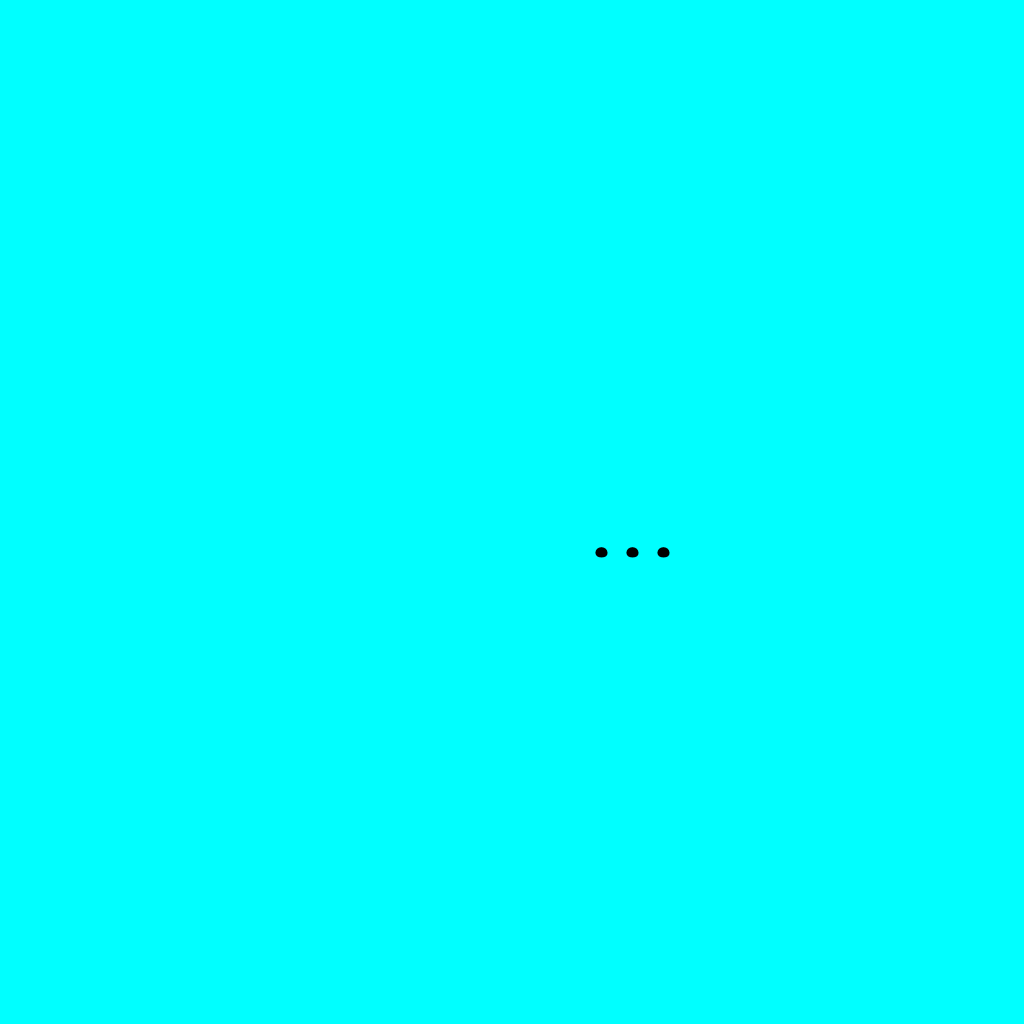func TruncateText(dc *gg.Context, originalText string, maxTextWidth float64) string {
tmpStr := ""
result := make([]rune, 0)
for _, r := range originalText {
tmpStr = tmpStr + string(r)
w, _ := dc.MeasureString(tmpStr)
if w > maxTextWidth {
if len(tmpStr) <= 1 {
return ""
} else {
break
}
} else {
result = append(result, r)
}
}
return string(result)
}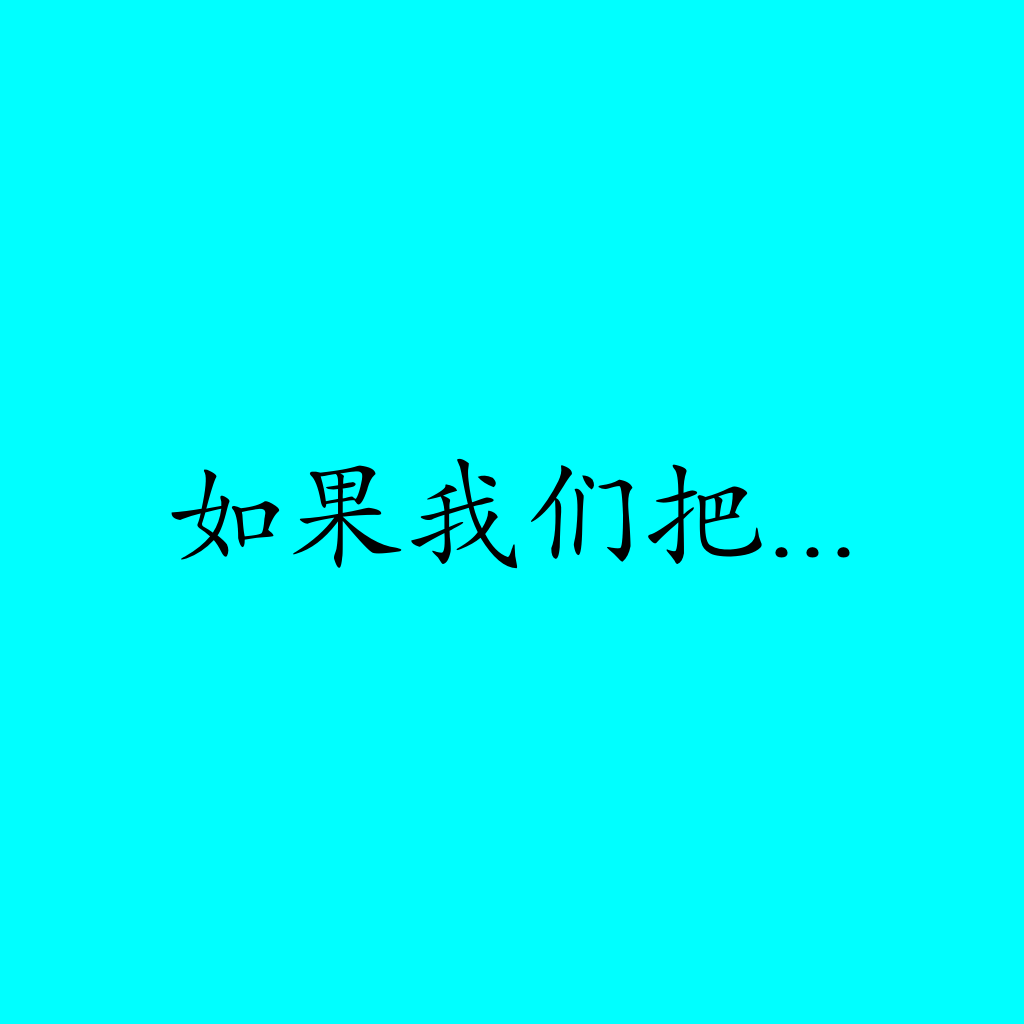多行文本处理

package main

import (
"github.com/fogleman/gg"
"strings"
)

func main() {
const S = 1024
dc := gg.NewContext(S, S)
dc.SetRGB(0, 1, 1)
dc.Clear()
dc.SetRGB(0, 0, 0)
panic(err)
}
s := "这是我的一个秘密，再简单不过的秘密：一个人只有用心去看，才能看到真实。事情的真相只用眼睛是看不见的。        --《小王子》"
ellipsisWidth, _ := dc.MeasureString("...")

maxTextWidth := S * 0.9
lineSpace := 25.0
maxLine := int(S / (dc.FontHeight() + lineSpace))

line := 0
lineTexts := make([]string, 0)
for len(s) > 0 {
line++
if line > maxLine {
break
}
if line == maxLine {
sw, _ := dc.MeasureString(s)
if sw > maxTextWidth {
maxTextWidth -= ellipsisWidth
}
}
lineText := TruncateText(dc, s, maxTextWidth)
if line == maxLine && len(lineText) < len(s) {
lineText += "..."
}
lineTexts = append(lineTexts, lineText)
if len(lineText) >= len(s) {
break
}
s = s[len(lineText):]
}

lineY := (S - dc.FontHeight()*float64(len(lineTexts)) - lineSpace*float64(len(lineTexts)-1)) / 2
lineY += dc.FontHeight()
for _, text := range lineTexts {
sWidth, _ := dc.MeasureString(text)
lineX := (S - sWidth) / 2
dc.DrawString(text, lineX, lineY)
lineY += dc.FontHeight() + lineSpace
}

dc.SavePNG("out.png")
}

func TruncateText(dc *gg.Context, originalText string, maxTextWidth float64) string {
tmpStr := ""
result := make([]rune, 0)
for _, r := range originalText {
tmpStr = tmpStr + string(r)
w, _ := dc.MeasureString(tmpStr)
if w > maxTextWidth {
if len(tmpStr) <= 1 {
return ""
} else {
break
}
} else {
result = append(result, r)
}
}
return string(result)
}

//如果已经是最后一行，则需要判断剩余字符串是否仍旧超过最大宽度
if line == maxLine {
sw, _ := dc.MeasureString(s)
// 如果超过则需要在末尾添加省略号，截取的最大宽度需要减去省略号的宽度
if sw > maxTextWidth {
maxTextWidth -= ellipsisWidth
}
}
lineText := TruncateText(dc, s, maxTextWidth)
// 如果是最后一行并且文字仍旧是被截取过，那么在末尾添加省略号
if line == maxLine && len(lineText) < len(s) {
lineText += "..."
}

lineY := (S - dc.FontHeight()*float64(len(lineTexts)) - lineSpace*float64(len(lineTexts)-1)) / 2

lineY += dc.FontHeight()
for _, text := range lineTexts {
sWidth, _ := dc.MeasureString(text)
lineX := (S - sWidth) / 2
dc.DrawString(text, lineX, lineY)
lineY += dc.FontHeight() + lineSpace
}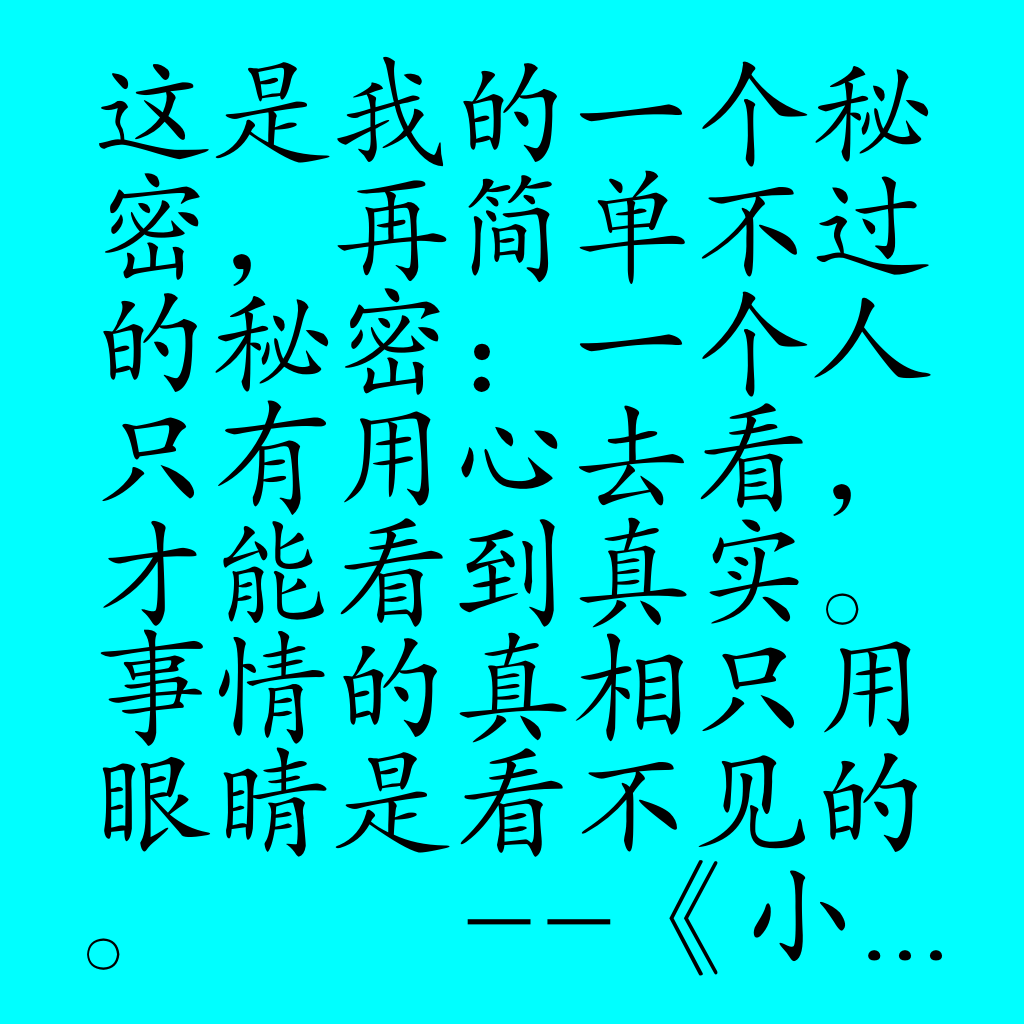小结posted @ 2020-12-20 10:34  弗兰克的猫  阅读(1401)  评论(0编辑  收藏  举报